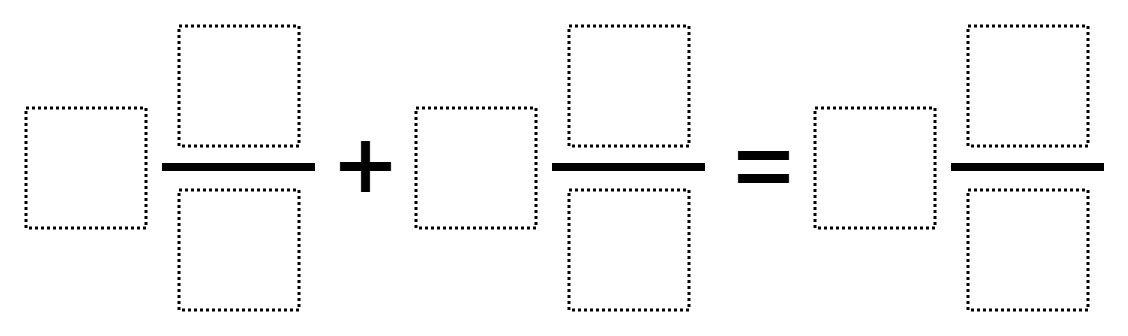Directions: Use the digits 1-9 each once to make a the largest possible sum.### Hint

What assumptions are we making about the fractions we are able to use? Do fractions have to be in lowest terms? How can we figure out if we want the larger numbers to be part of the addends or sum?

There are many answers in the comments below. One I got was 9 2/4 + 5 3/6 = 7 8/1

Source: Robert Kaplinsky and Ellen Metzger

## Equivalent Expressions with Fractions

Directions: Using the digits 0 to 9 at most one time each and choosing either …

1.This was a pretty tough problem. Had my class stumped. I eventually came up with 5 and 6/3 + 4 and 8/2 = 9 and 7/1. 9 and 7/1 = 16.

Thanks for the problem.

•Just realized my mistake after looking over my work. 6/3 is only 2, which would result in a total of 15 not 16. Back to the drawing board.

2.My students found this:

2 4/8 + 7 1/3 = 9 5/6

3.Without using improper fractions in your mixed numbers. We came up with 9 and 6/7 plus 8 and 4/5 which equals 18 and 23/35

4.I didn’t realize that the 1 to 9 rule also applied to the answer.

5.My students said “This problem is so intriguing!”
One of my 5th graders came up with:
5 4/6 + 7 1/3 = 9 8/2

6.My students found: 7 and 5/2 plus 1 and 3/6 which equals 9 and 8/4. They were pretty excited! I also added that now they needed to find another way, as perhaps a sum of 11 wasn’t the highest sum.

7.My students found 2 4/8 + 9 3/6 = 7 5/1

2 1/2 + 9 1/2 = 12

8.Hello. I am a student and was very stumped. But, I figured out that 2 4/8 plus 7 1/3 gets me 9 5/6.

9.8 6/4 + 5 3/2 = 9 7/1 = 16

10.11.Well i got 3 14/16 + 2 7/9 = 6 1/6 (simplest form)

12.